# Maharashtra Board Class 11 Chemistry Solutions Chapter 8 Elements of Group 1 and 2

Balbharti Maharashtra State Board 11th Chemistry Textbook Solutions Chapter 8 Elements of Group 1 and 2 Textbook Exercise Questions and Answers.

## Maharashtra State Board 11th Chemistry Solutions Chapter 8 Elements of Group 1 and 2

1. Explain the following

Question A.
Hydrogen shows similarity with alkali metals as well as halogens.

• Electronic configuration of hydrogen is 1s1 which is similar to the outer electronic configuration of alkali metals of group 1 i.e., ns1.
• However, 1s1 also resembles the outer electronic configuration of group 17 elements i.e., ns2 np5.
• By adding one electron to H, it will attain electronic configuration of the inert gas He which is 1s2 and by adding one electron to ns2 np5 we get ns2 np6 which is the outer electronic configuration of the remaining inert gases.
• Therefore, some chemical properties of hydrogen are similar to those of alkali metals while some resemble halogens.

Hence, hydrogen shows similarity with alkali metals as well as halogens.

Question B.
Standard reduction potential of alkali metals have high negative values.

• The general outer electronic configuration of alkali metals is ns1.
• They readily lose one valence shell electron to achieve stable noble gas configuration and hence, they are highly electropositive and are good reducing agents.

Hence, standard reduction potentials of alkali metals have high negative values.

Question C.
Alkaline earth metals have low values of electronegativity; which decrease down the group.

• Electronegativity represents attractive force exerted by the nucleus on shared electrons.
• The general outer electronic configuration of alkaline earth metals is ns2. They readily lose their two valence shell electrons to achieve stable noble gas configuration. They are electropositive and hence, they have low values of electronegativity.Question D.
Sodium dissolves in liquid ammonia to form a solution which shows electrical conductivity.
i. Sodium dissolves in liquid ammonia giving deep blue coloured solutions which is electrically conducting in nature.
Na + (x + y) NH3 → [Na(NH3)x]+ + [e(NH3)y]
ii. Due to formation of ions, the solution shows electrical conductivity.

Question E.
BeCl2 is covalent while MgCl2 is ionic.

• Be2+ ion has very small ionic size and therefore, it has very high charge density.
• Due to this, it has high tendency to distort the electron cloud around the negatively charged chloride ion (Cl) which is larger in size.
• This results in partial covalent character of the bond in BeCl2.
• Mg2+ ion has very less tendency to distort the electron cloud of Cl due to the bigger size of Mg2+ as compared to Be2+.

Hence, BeCl2 is covalent while MgCl2 is ionic.

Question F.
Lithium floats an water while sodium floats and catches fire when put in water.

• When lithium and sodium react with water, hydrogen gas is released. Due to these hydrogen gas bubbles, lithium and sodium floats on water.
eg. 2Na + 2H2O → 2Na+ + 2OH + H2
• The reactivity of group 1 metals increases with increasing atomic radius and lowering of ionization enthalpy down the group.
• Thus, sodium having lower ionization enthalpy, is more reactive than lithium.
• Hence, lithium reacts slowly while sodium reacts vigorously with water.
• Since the reaction of sodium with water is highly exothermic, it catches fire when put in water.2. Write balanced chemical equations for the following.

Question A.
CO2 is passed into concentrated solution of NaCl, which is saturated with NH3.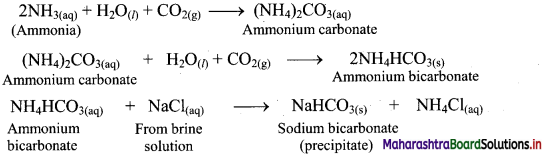Question B.
A 50% solution of sulphuric acid is subjected to electrolyte oxidation and the product is hydrolysed.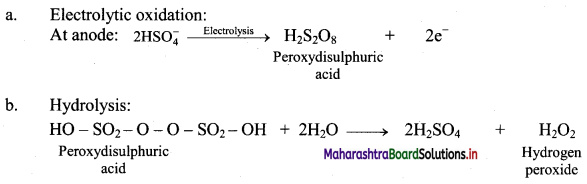Question C.
Magnesium is heated in air.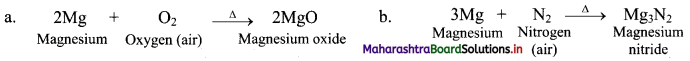Question D.
Beryllium oxide is treated separately with aqueous HCl and aqueous NaOH solutions.
Beryllium oxide (BeO) is an amphoteric oxide and thus, it reacts with both acid (HCl) as well as base (NaOH) to give the corresponding products.
i. $$\mathrm{BeO}+\underset{(\text { Acid })}{2 \mathrm{HCl}} \longrightarrow \mathrm{BeCl}_{2}+\mathrm{H}_{2} \mathrm{O}$$
ii. $$\mathrm{BeO}+\underset{(\text { Base })}{2 \mathrm{NaOH}} \longrightarrow \mathrm{Na}_{2} \mathrm{BeO}_{2}+\mathrm{H}_{2} \mathrm{O}$$Question A.
Describe the diagonal relationship between Li and Mg with the help of two illustrative properties.
a. The relative placement of these elements with similar properties in the periodic table is across a diagonal and is called diagonal relationship.
b. Lithium is placed in the group 1 and period 2 of the modem periodic table. It resembles with magnesium which is placed in the group 2 and period 3.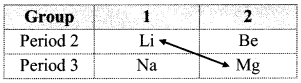ii. Li and Mg show similarities in many of their properties.
e. g.
a. Reaction with oxygen:
1. Group 1 elements except lithium, react with oxygen present in the air to form oxides (M2O) as well as peroxides (M2O2) and superoxides (MO2) on further reaction with excess of oxygen.
2. This anomalous behaviour of lithium is due to its resemblance with magnesium as a result of diagonal relationship.
3. As group 2 elements form monoxides i.e., oxides, lithium also form monoxides.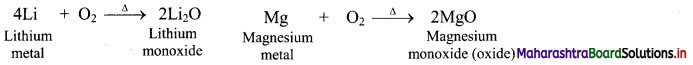b. Reaction with nitrogen:
1. All the group 1 elements react only with oxygen present in the air to form oxides while group 2 elements react with both nitrogen and oxygen present in the air forming corresponding oxides and nitrides.
2. However, lithium reacts with oxygen as well as nitrogen present in the air due to its resemblance with magnesium.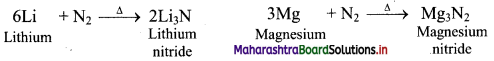Question B.
Describe the industrial production of dihydrogen from steam. Also write the chemical reaction involved.
Three stages are involved in the industrial production of dihydrogen from steam.
i. Stage 1:
a. Reaction of steam on hydrocarbon or coke (C) at 1270 K temperature in presence of nickel catalyst gives water-gas which is a mixture of carbon monoxide and hydrogen.
1. Reaction of steam with hydrocarbon: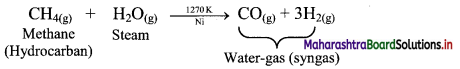2. Reaction of steam with coke or carbon (C):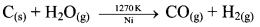b. Sawdust, scrapwood, etc. can also be used in place of carbon.

ii. Stage 2:
Water-gas shift reaction: When carbon monoxide in the water-gas reacts with steam in the presence of iron chromate (FeCrO4) as catalyst, it gets transformed into carbon dioxide. This is called water-gas shift reaction.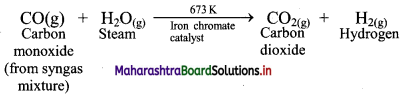iii. Stage 3: In the last stage, carbon dioxide is removed by scrubbing with sodium arsenite solution.

Question C.
A water sample, which did not give lather with soap, was found to contain Ca(HCO3)2 and Mg(HCO3)2. Which chemical will make this water give lather with soap? Explain with the help of chemical reactions.

• Soap does not lather in hard water due to presence of the soluble salts of calcium and magnesium in it. So, the given water sample is hard water.
• Hardness of hard water can be removed by removal of these calcium and magnesium salts.
• Sodium carbonate is used to make hard water soft as it precipitates out the soluble calcium and magnesium salts in hard water as carbonates. Thus, it will make water give lather with soap.
e.g. Ca(HCO3)2(aq) + Na2CO3(aq) → CaCO3(s) + 2NaHCO3(aq)Question D.
Name the isotopes of hydrogen. Write their atomic composition schematically and explain which of these is radioactive ?
i. Hydrogen has three isotopes i.e., hydrogen $$\left({ }_{1}^{1} \mathrm{H}\right)$$, deuterium $$\left({ }_{1}^{2} \mathrm{H}\right)$$ and tritium $$\left({ }_{1}^{3} \mathrm{H}\right)$$ with mass numbers 1, 2 and 3 respectively.
ii. They all contain one proton and one electron but different number of neutrons in the nucleus.
iii. Atomic composition of isotopes of hydrogen: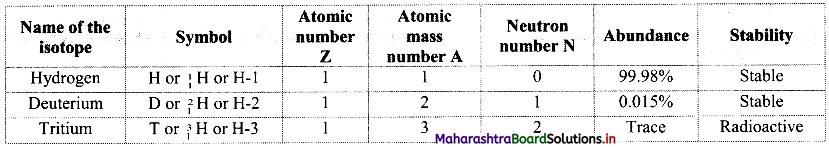iv. Tritium is a radioactive nuclide with half-life period 12.4 years and emits low energy β particles.
v. Schematic representation of isotopes of hydrogen is as follows: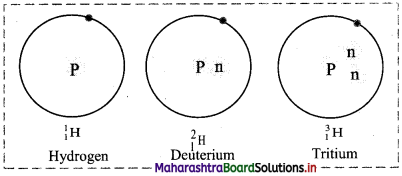4. Name the following

Question A.
Alkali metal with smallest atom.
Lithium (Li)

Question B.
The most abundant element in the universe.
Hydrogen (H)

Question C.
Francium (Fr)

Question D.
Ions having high concentration in cell sap.
Potassium ions (K+)

Question E.
A compound having hydrogen, aluminium and lithium as its constituent elements.
Lithium aluminium hydride (LiAlH4)5. Choose the correct option.

Question A.
The unstable isotope of hydrogen is …..
a. H-1
b. H-2
c. H-3
d. H-4
c. H-3

Question B.
Identify the odd one.
a. Rb
b. Ra
c. Sr
d. Be
a. Rb

Question C.
Which of the following is Lewis acid ?
a. BaCl2
b. KCl
c. BeCl2
d. LiCl
c. BeCl2

Question D.
What happens when crystalline Na2CO3 is heated ?
a. releases CO2
b. loses H2O
c. decomposes into NaHCO3
d. colour changes.
b. loses H2OActivity :

1. Collect the information of preparation of dihydrogen and make a chart.
2. Find out the s block elements compounds importance/uses.
1.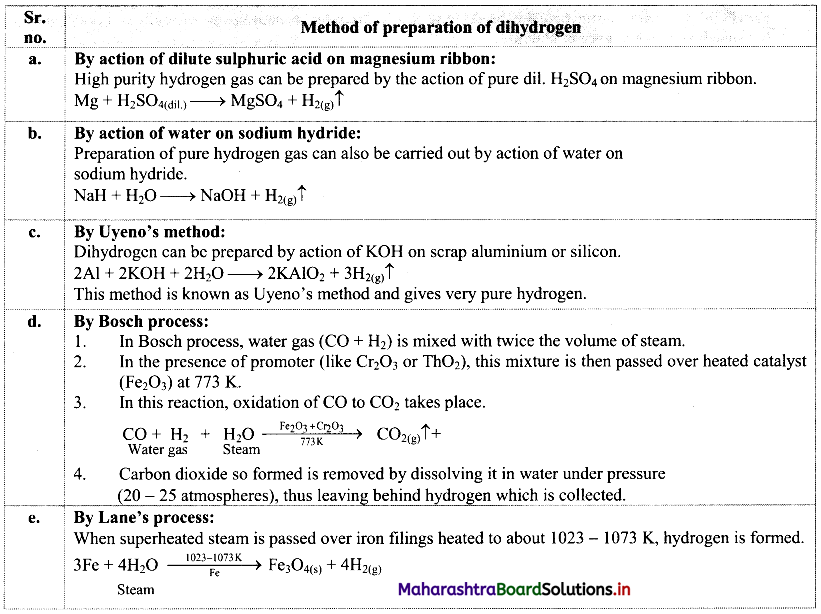2. Uses of s-block elements:
Group 1 elements (alkali metals):
a. Lithium: Lithium is widely used in batteries.
b. Sodium:

• Liquid sodium metal is used as a coolant in fast breeder nuclear reactors.
• Sodium is also used as an important reagent in the Wurtz reaction.
• It is used in the manufacture of sodium vapour lamp.

c. Potassium:

• Potassium has a vital role in biological system.
• Potassium chloride (KCl) is used as a fertilizer.
• Potassium hydroxide (KOH) is used in the manufacture of soft soaps and also as an excellent absorbent of carbon dioxide.
• Potassium superoxide (KO2) is used as a source of oxygen.

d. Caesium: Caesium is used in devising photoelectric cells.

Group 2 elements (alkaline earth metals):
a. Magnesium: Magnesium hydroxide [Mg(OH)2] in its suspension form is used as an antacid.
b. Calcium: Compounds of calcium such as limestone and gypsum are used as constituents of cement and mortar.
c. Barium: BaSO4 being insoluble in H2O and opaque to X-rays is used as ‘barium meal’ to scan the X-ray of human digestive system.
[Note: Students are expected to collect additional information about preparation of dihydrogen and uses of s-block elements on their own.]11th Chemistry Digest Chapter 8 Elements of Group 1 and 2 Intext Questions and Answers

Can you recall? (Textbook Page No. 110)

Question 1.
Which is the first element in the periodic table?
Hydrogen is the first element in the periodic table.

Question 2.
What are isotopes?
Many elements exist naturally as a mixture of two or more types of atoms or nuclides. These individual nuclides are called isotopes of that element. Isotopes of an element have the same atomic number (number of protons) but different atomic mass numbers due to different number of neutrons in their nuclei.

Question 3.
Write the formulae of the compounds of hydrogen formed with sodium and chlorine.
Hydrogen combines with sodium to form sodium hydride (NaH) while it reacts with chlorine to form hydrogen chloride (HCl).

Can you tell? (Textbook Page No. 110)

Question 1.
In which group should hydrogen be placed? In group 1 or group 17? Why?

• Hydrogen contains one valence electron in its valence shell and thus, its valency is one. Therefore, hydrogen resembles alkali metals (group 1 elements) as they also contain one electron in their valence shell (alkali metals tend to lose their valence electron).
• However, hydrogen also shows similarity with halogens (group 17 elements) as their valency is also one because halogens tend to accept one electron in their valence shell.
• Due to this unique behaviour, it is difficult to assign any definite position to hydrogen in the modem periodic table.Just think! (Textbook Page No. 112)

Question 1.
$$2 \mathrm{Na}_{(\mathrm{s})}+\mathrm{H}_{2(\mathrm{~g})} \stackrel{\Delta}{\longrightarrow} 2 \mathrm{NaH}_{(\mathrm{s})}$$
In the above chemical reaction which element does undergo oxidation and which does undergo reduction?
i. Redox reaction can be described as electron transfer as shown below:
2Na(s) + H2(g) → 2Na+ + 2H
ii. Charge development suggests that each sodium atom loses one electron to form Na+ and each hydrogen atom gains one electron to form H. This can be represented as follows: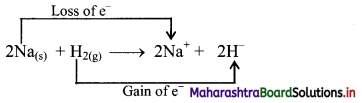iii. Na is oxidised to NaH by loss of electrons while the elemental hydrogen is reduced to NaH by gain of electrons.

Can you recall? (Textbook Page No. 113)

Question i.
What is the name of the family of reactive metals having valency one?